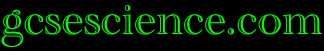CHEMISTRY Index
Moles

 Avogadro's Law Converting Relative Mass Avogadro's Number Concentrations Relative Atomic Mass Definition of a Mole Mass into Moles Relative Formula Mass Equations Moles into Mass Relative Molecular Mass Introduction Moles into Volume What is a Mole?

Calculations using Moles

1. The Mass of

 Atoms Compounds Molecules

2. The Mass of Products from Reactions.

 Basic Method Advanced Method MgO from Mg Basic Rules Advanced Rules Na2O from Na

3. The Percentage of an Element in a Compound.

 Method C in C3H6 Ca in CaCO3

4. The Empirical Formula from a Reaction.

 Empirical Formula Method Calcium Chloride Molecular Formula Iron Oxide

5. The Molecular Formula of Compounds.

 Method Propene Ethane

6. Volumes of Gases.

 Avogadro's Law CO2 from Carbon H2  from Zn in H2SO4 Method CO2 from Ethane Mass of Gas

7. Masses or Volumes from Electrolysis.

 Method Cl2  from NaCl(l) Pb from PbBr2 Amount of Electricity H2 from NaCl(aq) Al from Al2O3

8. Concentrations of Solutions.

To find a word on this page, press F3 on your keyboard and enter text into the box.